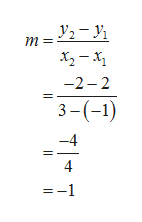Find an equation of a line passes through (-1,2) and (3,-2)

Question

Find an equation of a line passes through (-1,2) and (3,-2)

Step 1

The given points are represented as follows.

Step 2

Compute the slope of the line from the given points as follows.help_outlineImage Transcriptionclosem =2 _Ji -2- 2 3-(-1) -4 4 -1 fullscreen
Step 3

Consider the first point as x and y and obtain the y intercept b. (Choos...

Want to see the full answer?

See Solution

Want to see this answer and more?

Our solutions are written by experts, many with advanced degrees, and available 24/7

See Solution
Tagged in

Equations and In-equations